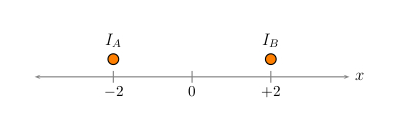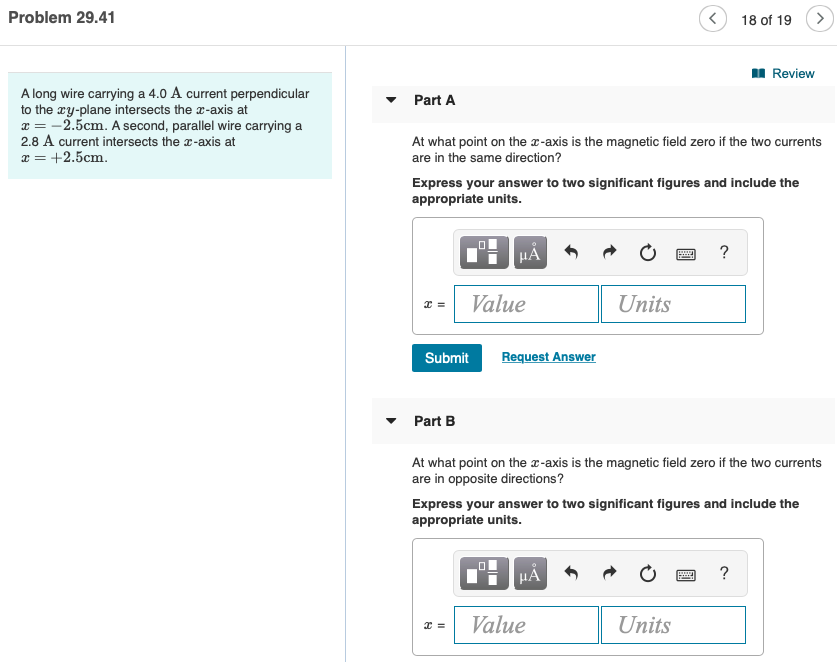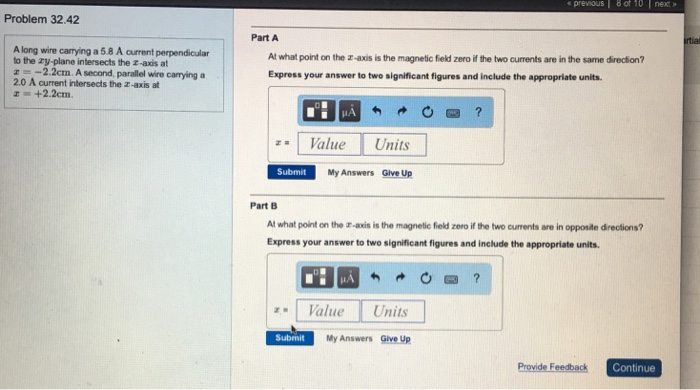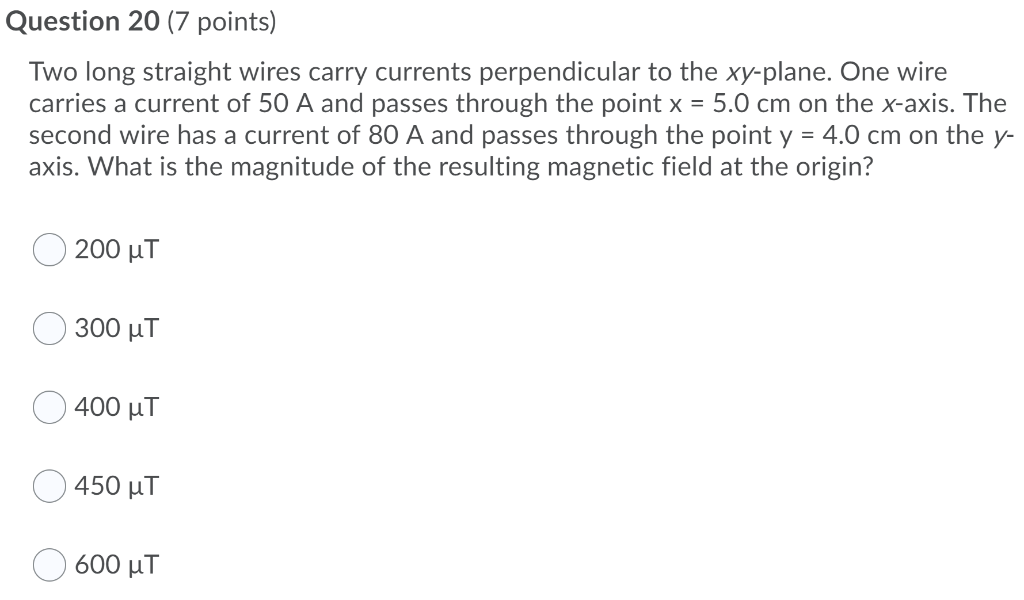# A long wire carrying a 5.0 A current perpendicular to the xy-plane intersects the x-axis at x =...

A long wire carrying a 5.0 A current perpendicular to the xy-plane intersects the x-axis at x = -2.00 cm. A second parallel wire carrying a 3.00 A current intersectsthe x-axis at x = +2.00 cm. At what point or points on the x-axis is the magnetic field zero (a) if the two currents are in the same direction and (b) if the twocurrents are in opposite directions?

This Homework Help Question: "A long wire carrying a 5.0 A current perpendicular to the xy-plane intersects the x-axis at x =..." No answers yet.

We need 9 more requests to produce the answer to this homework help question. Share with your friends to get the answer faster!

1 /10 have requested the answer to this homework help question.

Once 10 people have made a request, the answer to this question will be available in 1-2 days.
All students who have requested the answer will be notified once they are available.

#### Earn Coin

Coins can be redeemed for fabulous gifts.

Similar Homework Help Questions
• ### A long wire carrying a 4.6A current perpendicular to the xy-plane intersects the x-axis at x=-1.6cm....

A long wire carrying a 4.6A current perpendicular to the xy-plane intersects the x-axis at x=-1.6cm. A second, parallel wire carrying a 2.8A current intersects at x=+1.6cm. Part A At what point on the x-axis is the magnetic field zero if the two currents are in the same direction? Part B At what point on the x-axis is the magnetic field zero if the two currents are in opposite directions?

• ### A long wire carrying IA = 4.0 A  of current perpendicular to the xy-plane intersects the x-axis...A long wire carrying IA = 4.0 A  of current perpendicular to the xy-plane intersects the x-axis at x=−2.0cm . A second, parallel wire carrying IB = 3.5 A  of current intersects the x-axis at x=+2.0cm . (As illustrated in the figure, the two currents are either into or out of the page.) At what point on the x-axis is the magnetic field zero if the two currents are in opposite directions? IA +2 B 2

• ### Wires carrying a current

A long wire carrying a 5.5 A current perpendicular to the xy-plane intersects the x-axis at x=-2.0cm. A second, parallel wire carrying a 3.0 A current intersects thex-axis at x=+2.0cm.At what point on the x-axis is the magnetic field zero if the two currents are in the same direction?At what point on the x-axis is the magnetic field zero if the two currents are in opposite directions?

• ### Magnetic field and current question. Will rate HIGH for correct answer w/ explanation!

A long wire carrying a 4.5 A current perpendicular to the xy-plane intersects the x-axis at x= -2.0 cm. A second, parallel wire carrying a 3.0 A current intersects thex-axis at x= +2.0 cm.1. At what point on the x-axis is the magnetic field zero if the two currents are in the same direction? (in cm)2. At what point on the x-axis is the magnetic field zero if the two currents are in opposite directions? (in cm)Thank you!

• ### What point on an X axis is the magnetic field zero if the currents are in opposite directions?

A long wire carrying a 5.5 A current perpendicular to the xy-plane intersects the x-axis at x=- 2.0 cm . A second, parallel wire carrying a 2.5 A current intersectsthe x-axis at x= 2.0 cm.At what point on the x-axis is the magnetic field zero if the two currents are in opposite directions?

• ### What point on an X axis is the magnetic field zero?

A long wire carrying a 5.5 A current perpendicular to the xy-plane intersects the x-axis at x,=: -, 2.0;{rm cm} . A second, parallel wire carrying a 2.5 A currentintersects the x-axis at x,=:+; 2.0;{rm cm} .At what point on the x-axis is the magnetic field zero if the two currents are in the same direction?

• ### Problem 29.41 18 of 19 > Review Part A Along wire carrying a 4.0 A current...Problem 29.41 18 of 19 > Review Part A Along wire carrying a 4.0 A current perpendicular to the cy-plane intersects the x-axis at = -2.5cm. A second, parallel wire carrying a 2.8 A current intersects the 3-axis at = +2.5cm. At what point on the 2-axis is the magnetic field zero if the two currents are in the same direction? Express your answer to two significant figures and include the appropriate units. OTH HÅR ? Value Units Submit Request...

• ### previous 8 of 10 nextョ Problem 32.42 Part A tial A long wire carrying a 5.8...previous 8 of 10 nextョ Problem 32.42 Part A tial A long wire carrying a 5.8 A current perpendicular to the zy-plane intersects the z-axis at r-2.2cm. A second, parallel wire carrying a 2.0 A current intersects the z-axis at z +2.2cm At what point on the x-axis is the magnetic fiekd zero if the two currents are in the same direction? Express your answer to two significant figures and include the appropriate units. Value Units Submit My Answers Give...

• ### Question 20 (7 points) Two long straight wires carry currents perpendicular to the xy-plane. One wire...Question 20 (7 points) Two long straight wires carry currents perpendicular to the xy-plane. One wire carries a current of 50 A and passes through the point x = 5.0 cm on the x-axis. The second wire has a current of 80 A and passes through the point y = 4.0 cm on the y- axis. What is the magnitude of the resulting magnetic field at the origin? 200 ut 300 uT 400 uT 450 uT 600 uT

• ### 1. A long straight wire lies on the x-axis and carries a current. On the positive...

1. A long straight wire lies on the x-axis and carries a current. On the positive y-axis, the magnetic field points in the negative z direction. What direction does the current in the wire run? (a) Positive x direction. (b) Negative x direction. (c) The current is zero. (d) More information is required. 2. What is the Biot-Savart Law? (a) A statement of the magnetic field due to a segment of current carrying wire. (b) A statement of the magnetic...